Anzeige

# 555 timer vibrators

10. Sep 2020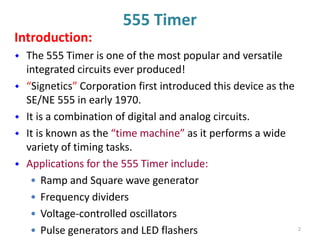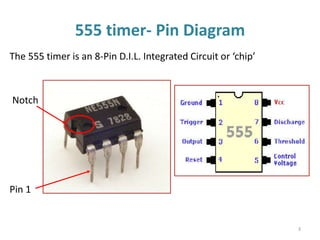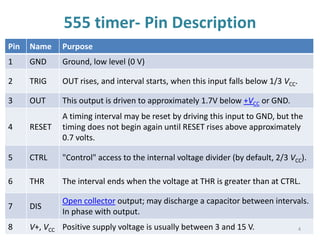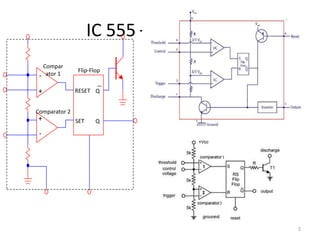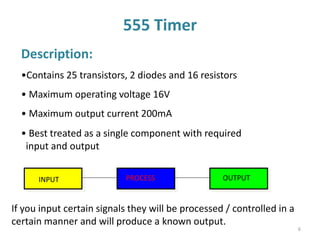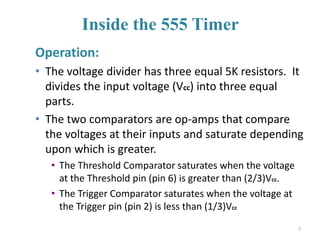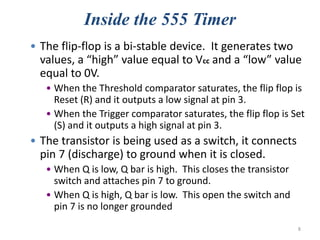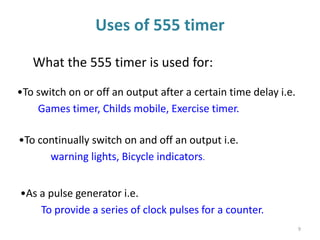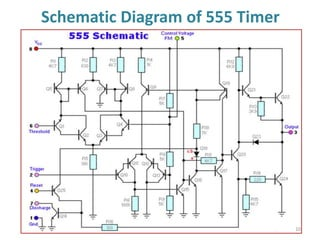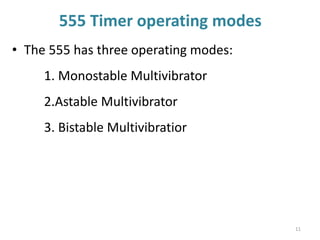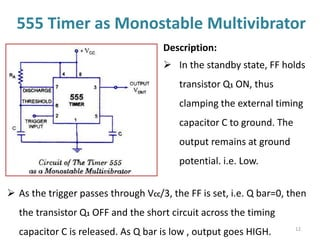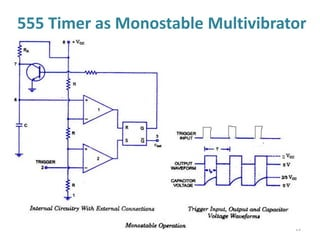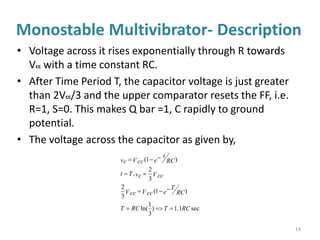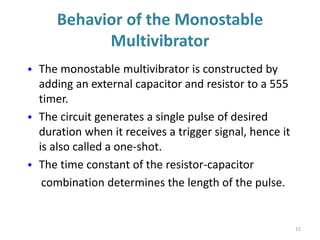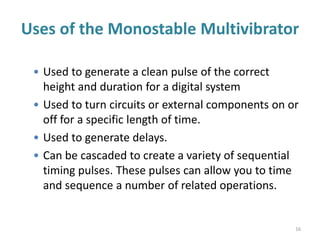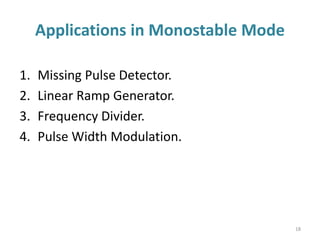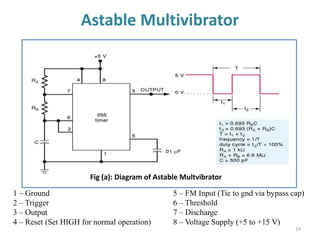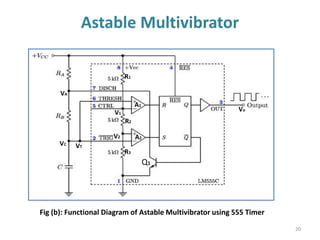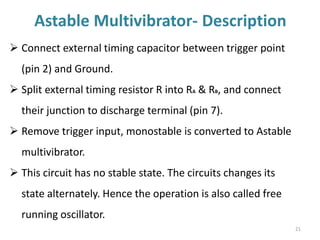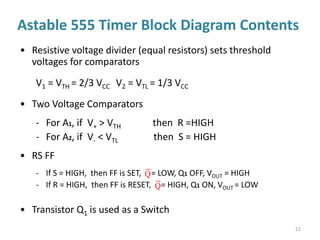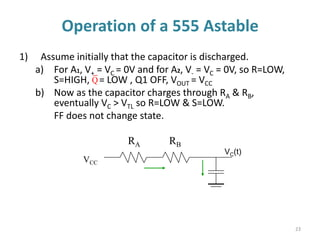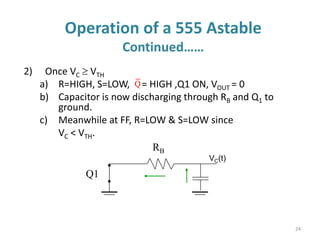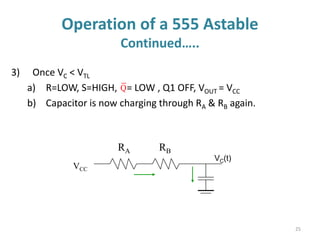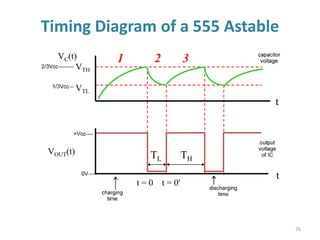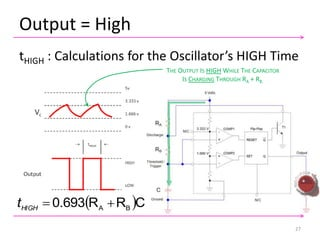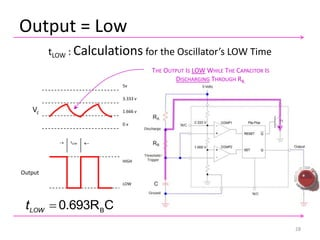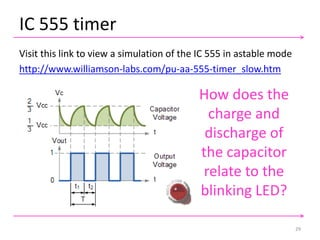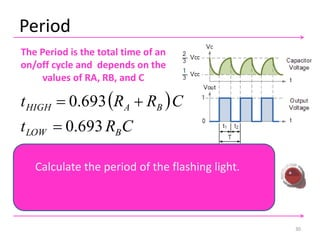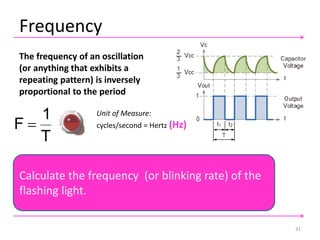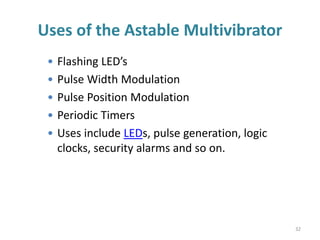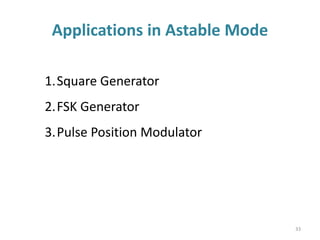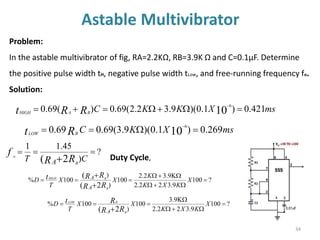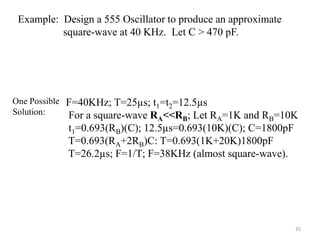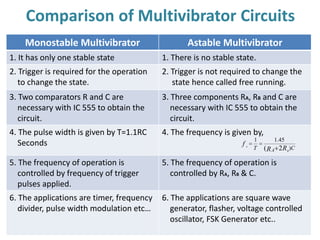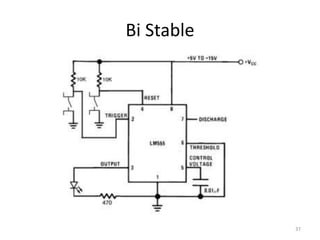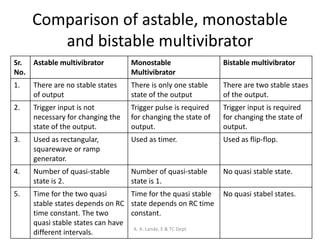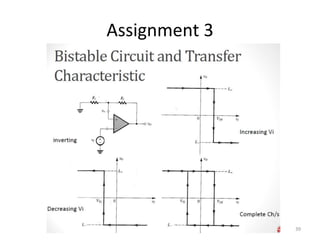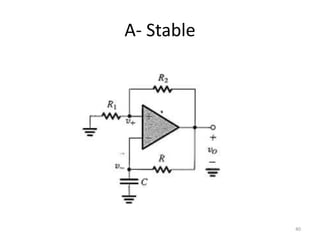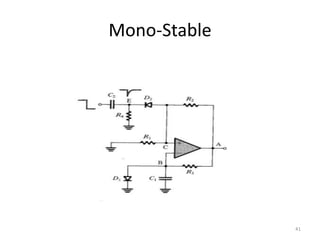1 von 41
Anzeige

### 555 timer vibrators

1. 555 TIMER Multivibtrator 1
2. 555 Timer Introduction:  The 555 Timer is one of the most popular and versatile integrated circuits ever produced!  “Signetics” Corporation first introduced this device as the SE/NE 555 in early 1970.  It is a combination of digital and analog circuits.  It is known as the “time machine” as it performs a wide variety of timing tasks.  Applications for the 555 Timer include: • Ramp and Square wave generator • Frequency dividers • Voltage-controlled oscillators • Pulse generators and LED flashers 2
3. 555 timer- Pin Diagram The 555 timer is an 8-Pin D.I.L. Integrated Circuit or ‘chip’ Notch Pin 1 3
4. 555 timer- Pin Description Pin Name Purpose 1 GND Ground, low level (0 V) 2 TRIG OUT rises, and interval starts, when this input falls below 1/3 VCC. 3 OUT This output is driven to approximately 1.7V below +VCC or GND. 4 RESET A timing interval may be reset by driving this input to GND, but the timing does not begin again until RESET rises above approximately 0.7 volts. 5 CTRL "Control" access to the internal voltage divider (by default, 2/3 VCC). 6 THR The interval ends when the voltage at THR is greater than at CTRL. 7 DIS Open collector output; may discharge a capacitor between intervals. In phase with output. 8 V+, VCC Positive supply voltage is usually between 3 and 15 V. 4
5. IC 555 – Why 555? 5 - + - + RESET SET Q Q Compar ator 1 Comparator 2 Flip-Flop
6. 555 Timer Description: •Contains 25 transistors, 2 diodes and 16 resistors • Maximum operating voltage 16V • Maximum output current 200mA If you input certain signals they will be processed / controlled in a certain manner and will produce a known output. INPUT PROCESS OUTPUT • Best treated as a single component with required input and output 6
7. Inside the 555 Timer Operation: • The voltage divider has three equal 5K resistors. It divides the input voltage (Vcc) into three equal parts. • The two comparators are op-amps that compare the voltages at their inputs and saturate depending upon which is greater. • The Threshold Comparator saturates when the voltage at the Threshold pin (pin 6) is greater than (2/3)Vcc. • The Trigger Comparator saturates when the voltage at the Trigger pin (pin 2) is less than (1/3)Vcc 7
8. Inside the 555 Timer • The flip-flop is a bi-stable device. It generates two values, a “high” value equal to Vcc and a “low” value equal to 0V. • When the Threshold comparator saturates, the flip flop is Reset (R) and it outputs a low signal at pin 3. • When the Trigger comparator saturates, the flip flop is Set (S) and it outputs a high signal at pin 3. • The transistor is being used as a switch, it connects pin 7 (discharge) to ground when it is closed. • When Q is low, Q bar is high. This closes the transistor switch and attaches pin 7 to ground. • When Q is high, Q bar is low. This open the switch and pin 7 is no longer grounded 8
9. Uses of 555 timer What the 555 timer is used for: •To switch on or off an output after a certain time delay i.e. Games timer, Childs mobile, Exercise timer. •To continually switch on and off an output i.e. warning lights, Bicycle indicators. •As a pulse generator i.e. To provide a series of clock pulses for a counter. 9
10. Schematic Diagram of 555 Timer 10
11. 555 Timer operating modes • The 555 has three operating modes: 1. Monostable Multivibrator 2.Astable Multivibrator 3. Bistable Multivibratior 11
12. 555 Timer as Monostable Multivibrator Description:  In the standby state, FF holds transistor Q1 ON, thus clamping the external timing capacitor C to ground. The output remains at ground potential. i.e. Low.  As the trigger passes through VCC/3, the FF is set, i.e. Q bar=0, then the transistor Q1 OFF and the short circuit across the timing capacitor C is released. As Q bar is low , output goes HIGH. 12
13. 555 Timer as Monostable Multivibrator 13
14. Monostable Multivibrator- Description • Voltage across it rises exponentially through R towards Vcc with a time constant RC. • After Time Period T, the capacitor voltage is just greater than 2Vcc/3 and the upper comparator resets the FF, i.e. R=1, S=0. This makes Q bar =1, C rapidly to ground potential. • The voltage across the capacitor as given by, sec1.1) 3 1 ln( )1( 3 2 3 2 , )1( RCTRCT e RC T V ccV cc V ccvcTt e RC t V ccvc     14
15. Behavior of the Monostable Multivibrator  The monostable multivibrator is constructed by adding an external capacitor and resistor to a 555 timer.  The circuit generates a single pulse of desired duration when it receives a trigger signal, hence it is also called a one-shot.  The time constant of the resistor-capacitor combination determines the length of the pulse. 15
16. Uses of the Monostable Multivibrator • Used to generate a clean pulse of the correct height and duration for a digital system • Used to turn circuits or external components on or off for a specific length of time. • Used to generate delays. • Can be cascaded to create a variety of sequential timing pulses. These pulses can allow you to time and sequence a number of related operations. 16
17. Monostable Multivibrator 17 F xx x R T C 9.0 1001.1 100 1.1 10 10 3 3    Problem: In the monostable multivibrator of fig, R=100kΩ and the time delay T=100ms. Calculate the value of C ? Solution: T=1.1RC
18. Applications in Monostable Mode 1. Missing Pulse Detector. 2. Linear Ramp Generator. 3. Frequency Divider. 4. Pulse Width Modulation. 18
19. Astable Multivibrator 19 1 – Ground 5 – FM Input (Tie to gnd via bypass cap) 2 – Trigger 6 – Threshold 3 – Output 7 – Discharge 4 – Reset (Set HIGH for normal operation) 8 – Voltage Supply (+5 to +15 V) Fig (a): Diagram of Astable Multvibrator
20. Astable Multivibrator 20 Fig (b): Functional Diagram of Astable Multivibrator using 555 Timer A1 A2 V1 V2 VT VC Vo VA R2 R1 R3 A1 A2 Q1
21. Astable Multivibrator- Description 21  Connect external timing capacitor between trigger point (pin 2) and Ground.  Split external timing resistor R into RA & RB, and connect their junction to discharge terminal (pin 7).  Remove trigger input, monostable is converted to Astable multivibrator.  This circuit has no stable state. The circuits changes its state alternately. Hence the operation is also called free running oscillator.
22. 22 • Resistive voltage divider (equal resistors) sets threshold voltages for comparators V1 = VTH = 2/3 VCC V2 = VTL = 1/3 VCC • Two Voltage Comparators - For A1, if V+ > VTH then R =HIGH - For A2, if V- < VTL then S = HIGH • RS FF - If S = HIGH, then FF is SET, = LOW, Q1 OFF, VOUT = HIGH - If R = HIGH, then FF is RESET, = HIGH, Q1 ON, VOUT = LOW • Transistor Q1 is used as a Switch Astable 555 Timer Block Diagram Contents Q Q
23. 23 Operation of a 555 Astable VCC VC(t) RA RB 1) Assume initially that the capacitor is discharged. a) For A1, V+ = VC = 0V and for A2, V- = VC = 0V, so R=LOW, S=HIGH, = LOW , Q1 OFF, VOUT = VCC b) Now as the capacitor charges through RA & RB, eventually VC > VTL so R=LOW & S=LOW. FF does not change state. Q
24. 24 Operation of a 555 Astable Continued…… VC(t) RB Q1 2) Once VC  VTH a) R=HIGH, S=LOW, = HIGH ,Q1 ON, VOUT = 0 b) Capacitor is now discharging through RB and Q1 to ground. c) Meanwhile at FF, R=LOW & S=LOW since VC < VTH. Q
25. 25 Operation of a 555 Astable Continued….. 3) Once VC < VTL a) R=LOW, S=HIGH, = LOW , Q1 OFF, VOUT = VCC b) Capacitor is now charging through RA & RB again. VCC VC(t) RA RB Q
26. Timing Diagram of a 555 Astable 26 VC(t) VTH VTL VOUT(t) TL TH t = 0 t = 0' t t 1 2 3
27. Output = High 27 tHIGH : Calculations for the Oscillator’s HIGH Time THE OUTPUT IS HIGH WHILE THE CAPACITOR IS CHARGING THROUGH RA + RB.  CRR0.693 BA HIGH t
28. 28 C0.693RB LOW t tLOW : Calculations for the Oscillator’s LOW Time 5v 3.333 v Vc 1.666 v 0 v  tLOW  Output HIGH LOW THE OUTPUT IS LOW WHILE THE CAPACITOR IS DISCHARGING THROUGH RB. Output = Low
29. IC 555 timer 29 Visit this link to view a simulation of the IC 555 in astable mode http://www.williamson-labs.com/pu-aa-555-timer_slow.htm How does the charge and discharge of the capacitor relate to the blinking LED?
30. Period The Period is the total time of an on/off cycle and depends on the values of RA, RB, and C 30         C2693.0 693.0693.0 693.0 693.0 BA BBA LOWHIGH BLOW BAHIGH RRT CRCRRT ttT CRt CRRt      Calculate the period of the flashing light.
31. Frequency The frequency of an oscillation (or anything that exhibits a repeating pattern) is inversely proportional to the period  CR2R693.0 1 F T 1 F BA    31 Calculate the frequency (or blinking rate) of the flashing light. Unit of Measure: cycles/second = Hertz (Hz)
32. Uses of the Astable Multivibrator • Flashing LED’s • Pulse Width Modulation • Pulse Position Modulation • Periodic Timers • Uses include LEDs, pulse generation, logic clocks, security alarms and so on. 32
33. Applications in Astable Mode 33 1.Square Generator 2.FSK Generator 3.Pulse Position Modulator
34. Astable Multivibrator 34 Problem: In the astable multivibrator of fig, RA=2.2KΩ, RB=3.9K Ω and C=0.1µF. Determine the positive pulse width tH, negative pulse width tLow, and free-running frequency fo. Solution: msXKCRt BLOW 269.0)1.0)(9.3(69.069.0 10 6   ? ) 45.11 2(   CT RR f A B o msXKKCRRt BAHIGH 421.0)1.0)(9.32.2(69.0)(69.0 10 6   ?100 9.322.2 9.32.2 100 ) ) 100% 2( (       X KXK K XX T D RR RRt A A B BHIGH Duty Cycle, ?100 9.322.2 9.3 100 ) 100% 2(      X KXK XX T D RR Rt A B BLOW
35. 35 One Possible Solution: Example: Design a 555 Oscillator to produce an approximate square-wave at 40 KHz. Let C > 470 pF. F=40KHz; T=25µs; t1=t2=12.5µs For a square-wave RA<<RB; Let RA=1K and RB=10K t1=0.693(RB)(C); 12.5µs=0.693(10K)(C); C=1800pF T=0.693(RA+2RB)C: T=0.693(1K+20K)1800pF T=26.2µs; F=1/T; F=38KHz (almost square-wave).
36. Comparison of Multivibrator Circuits 36 Monostable Multivibrator Astable Multivibrator 1. It has only one stable state 1. There is no stable state. 2. Trigger is required for the operation to change the state. 2. Trigger is not required to change the state hence called free running. 3. Two comparators R and C are necessary with IC 555 to obtain the circuit. 3. Three components RA, RB and C are necessary with IC 555 to obtain the circuit. 4. The pulse width is given by T=1.1RC Seconds 4. The frequency is given by, 5. The frequency of operation is controlled by frequency of trigger pulses applied. 5. The frequency of operation is controlled by RA, RB & C. 6. The applications are timer, frequency divider, pulse width modulation etc… 6. The applications are square wave generator, flasher, voltage controlled oscillator, FSK Generator etc.. CT RR f A B o ) 45.11 2(  
37. Bi Stable 37
38. Comparison of astable, monostable and bistable multivibrator Sr. No. Astable multivibrator Monostable Multivibrator Bistable multivibrator 1. There are no stable states of output There is only one stable state of the output There are two stable staes of the output. 2. Trigger input is not necessary for changing the state of the output. Trigger pulse is required for changing the state of output. Trigger input is required for changing the state of output. 3. Used as rectangular, squarewave or ramp generator. Used as timer. Used as flip-flop. 4. Number of quasi-stable state is 2. Number of quasi-stable state is 1. No quasi stable state. 5. Time for the two quasi stable states depends on RC time constant. The two quasi stable states can have different intervals. Time for the quasi stable state depends on RC time constant. No quasi stabel states. A. A. Lande, E & TC Dept
39. Assignment 3 39
40. A- Stable 40
41. Mono-Stable 41

### Hinweis der Redaktion

1. Derivation of the equation for t-LOW.
Anzeige